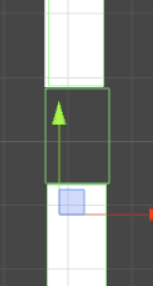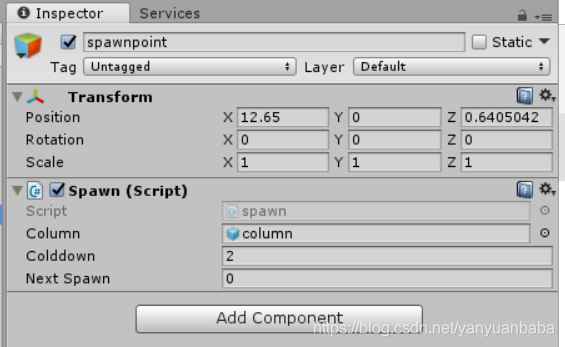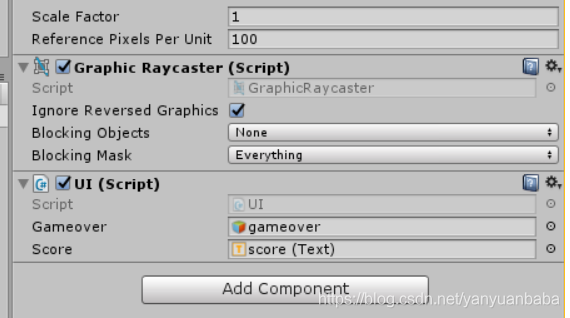|     |   0 浏览

## 制作柱体## bird的script

``````using System.Collections;
using System.Collections.Generic;
using UnityEngine;

public class birdcontrol : MonoBehaviour
{

Rigidbody2D bird;

public float speed;                                         //使用speed方便在项目里面选择一个合适的倍数

// Use this for initialization
void Start()                                                //游戏一开始就会调用的函数
{
bird = GetComponent<Rigidbody2D>();                     //将游戏里面的bird引入
}
// Update is called once per frame
void Update()                                               //游戏每一帧都会调用的函数
{
if (Input.GetKeyDown("space"))
{
bird.AddForce(new Vector2(0, 2 * speed));           //vector2相当于一个有xy的矢量
}
}
}
``````

## column的sprite

``````using System.Collections;
using System.Collections.Generic;
using UnityEngine;

public class columncontrol : MonoBehaviour {
public float speed;
// Use this for initialization
void Start () {

}

// Update is called once per frame
void Update () {
this.transform.Translate(new Vector2(-speed,0));
}

private void OnTriggerExit2D(Collider2D collision)
{
if (collision.gameObject.tag == "player")
{
Debug.Log("1");
}
}
}
``````

## column的生成

``````using System.Collections;
using System.Collections.Generic;
using UnityEngine;

public class spawn : MonoBehaviour {

public GameObject column;
public float colddown = 2f;
public float NextSpawn;
// Use this for initialization
void Start () {

}

// Update is called once per frame
void Update () {
if (Time.time > NextSpawn)
{
NextSpawn = Time.time + colddown;

Vector3 spawnP = transform.position;
spawnP.y += Random.Range(3.0f, -3.0f);
Instantiate(column, spawnP,transform.rotation);
}
}
}
``````## 游戏判定

``````using System.Collections;
using System.Collections.Generic;
using UnityEngine;
//GM.cs
public class GM : MonoBehaviour {

public static int score = 0;

public static bool Active = true;

// Use this for initialization
void Start () {

}

// Update is called once per frame
void Update () {

}
}
``````
``````using System.Collections;
using System.Collections.Generic;
using UnityEngine;
//hitted.cs
public class Hitted : MonoBehaviour {

// Use this for initialization
void Start () {

}

// Update is called once per frame
void Update () {

}

private void OnCollisionEnter2D(Collision2D collision)
{
if (collision.gameObject.tag == "Player")
{
GM.Active = false;
}
}
}
``````

``````if (GM.Active)		//columncontrol.cs
{
this.transform.Translate(new Vector2(-speed, 0));
}
1234
``````
``````if (Time.time > NextSpawn&&GM.Active)	//spawn.cs
{
NextSpawn = Time.time + colddown;

Vector3 spawnP = transform.position;
spawnP.y += Random.Range(3.0f, -3.0f);
Instantiate(column, spawnP,transform.rotation);
}
``````

## 游戏结束画面

``````using System.Collections;
using System.Collections.Generic;
using UnityEngine;
using UnityEngine.UI;

public class UI : MonoBehaviour {
public GameObject gameover;
public Text score;
// Use this for initialization
void Start () {

}

// Update is called once per frame
void Update () {

score.text = "Score" + GM.score;

if (!GM.Active)
{
gameover.SetActive(true);		//这里会让gameover显示
}
}
}
``````

UI.cs要添加到canvas上并在相应的位置把score和gameover应用上去。## 结语

-----------------------------